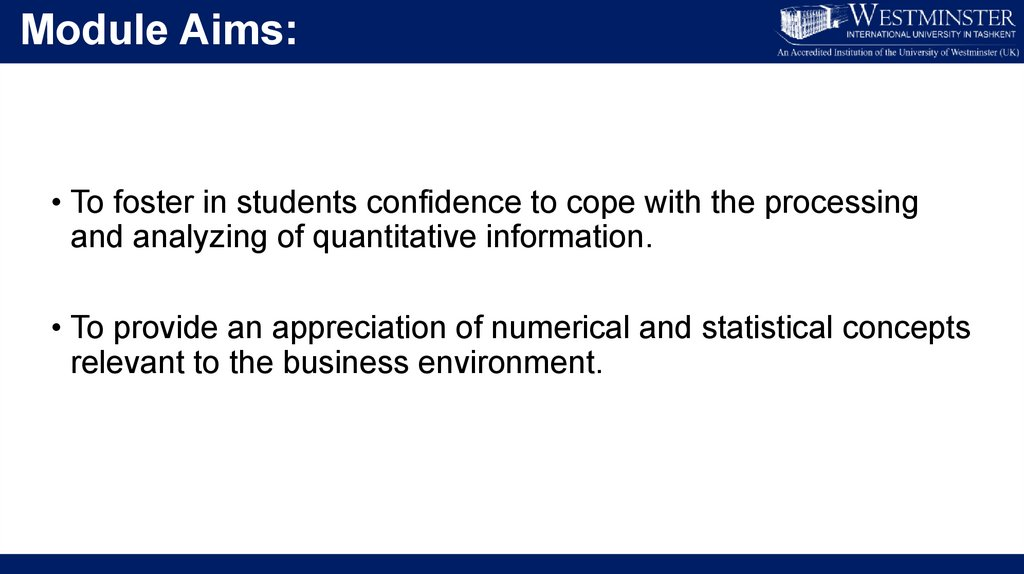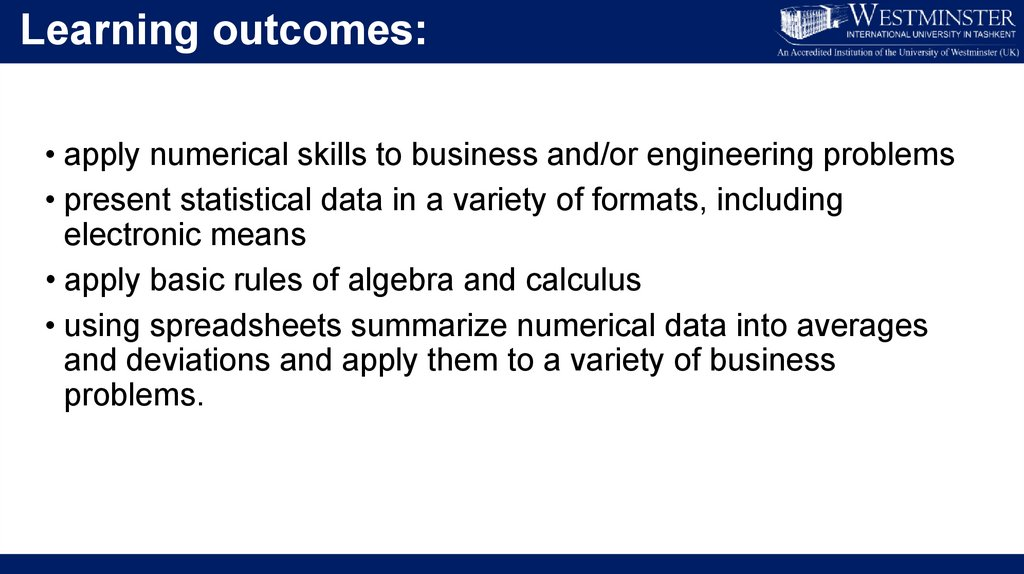# Data and data representation (lecture 1)

## 2.

Module Aims:
• To foster in students confidence to cope with the processing
and analyzing of quantitative information.
• To provide an appreciation of numerical and statistical concepts

## 3.

Learning outcomes:
• apply numerical skills to business and/or engineering problems
• present statistical data in a variety of formats, including
electronic means
• apply basic rules of algebra and calculus
• using spreadsheets summarize numerical data into averages
and deviations and apply them to a variety of business
problems.

## 4.

In brief, you will learn how ...:
• To appreciate benefit of numerical data for businesses
• To make decisions based on the numerical data
• To interpret and represent numerical data in a most appropriate
• To solve statistics and calculus problems using various
quantitative methods
• Note: You can find out more about module content in module
syllabus and 12-week teaching schedule.

## 5.

Teaching methods:
• 1-hour online lecture each week (online)
• 2-hour tutorial each week (offline)
• 1-hour workshop each week (offline)
You will learn the theory and its application

## 6.

Assessment methods:
Two assessments (or components):
• In-class test (30%+10%).
• 30% goes to an in-class test in Teaching Week 6
• 10% goes to weekly online mini-quizzes
• Final exam (60%) in Final exam week
• True/false
• Theory description
• Problem solving
• Open ended questions
• Multiple choice

## 7.

LECTURE 1
DATA & DATA REPRESENTATION
Temur Makhkamov
[email protected]
[email protected]
Office hours: by appointment
Room IB 205
EXT: 546

## 8.

Lecture outline
DATA
the meaning and types of data
sources of data
the scales of measurements for data
DATA REPRESENTATION TECHNIQUES AND TOOLS
analyze the quantitative and qualitative data;
display data in the form of table;
display data in the form of graph.

## 9.

What is data? (1)
• Data –
• the facts and figures that are collected, analyzed and summarized.
Examples: data about people, countries, employees
nature, universities, number of products sold, costs, prices,
movies, cars, hospitals, registration numbers, tax codes etc

## 10.

What is data? (2)
• Data may be obtained through already existing-sources or through
statistical studies.
Salaries, sales, advertising costs, inventory levels can be
disclosed from a company,
2. from a statistical study:
an experiment, a questionnaire, a survey, etc

## 11.

Primary and Secondary data
• Primary data – the data that are obtained as a result of
conducting a questionnaire, a survey, an interview, an observation,
etc.
Examples:__________________________________________
• Secondary data – the data that come from existing sources.
Government institutions, healthcare facilities, Internet and others
can provide a great deal of information in a ready-to-estimate
format.
Examples:__________________________________________

## 12.

Questions:
What data is more costly (expensive):
primary or secondary?
What data is more reliable (trustworthy):
primary or secondary?

## 13.

Statistical data
Q: What are the components of the statistical table?

## 14.

Components of the tabular data
• Element – the entity or item on which data are collected.
Examples: Westminster College, Yale Univ., etc
• Variable – a characteristic of interest for an element.
Examples: Enrollment, type, etc
• Observation – a set of measurements collected for a particular
element.
Examples: 953, coed, public, \$6,140, etc

## 15.

Main types of data
• Qualitative data provide labels or names for variables. They can
be nonnumeric descriptions or numeric codes.
Examples: Coed, Public, etc
• Quantitative data show an amount of variables. They indicate
either “how much” or “how many” of something.
Examples: 953 students, \$6,140 for Room & Boarding, etc

## 16.

Question:
• Consider this room as an element.
Are its variables such as,
Names of students
Mode of students
Number of students
quantitative or qualitative?
quantitative or qualitative?
quantitative or qualitative?

## 17.

Quantitative Data
Quantitative
Data
Discrete
Continuous
• Discrete data – the data obtained as a result of counting.
Examples: Number of enrolled students: 500, 1000, 2458, etc.
• Continuous data – the data that can take any value within a
continuum, limited only by the precision of the measurement
instrument.
Examples: Length or height of some object: 5 cm, 5.35 cm,

## 18.

Scale of Measurement

## 19.

SM for Qualitative Data (1)
• Nominal scale – a scale of measurement that uses name or label
to define a characteristic of an element.

## 21.

SM for Qualitative Data (2)
• Ordinal scale – a scale of measurement that is nominal and
allows ranking or ordering the data according to some criteria.

## 23.

SM for Quantitative Data (1)
• Interval scale – a scale of measurement that is ordinal and
intervals between data can be used to compare variable
observations.

## 25.

SM for Quantitative Data (2)
• Ratio scale – a scale of measurement that is interval and allows
considering the ratio of two data values.

## 29.

Raw data
• Raw data – the data that has not been processed (analyzed,
categorized, put in a table) yet.
Example:
Number of students (total 100), who attended 12 lectures: 100, 98,
85, 76, 64, 55, 76, 87, 96, 98, 99 & 100

## 30.

Aggregate data
• Aggregate data – the data that has already been processed to
serve one’s goal.
Example:
On four lectures, the attendance of students was lower than 80
and on other eight lectures it was greater or equal to 80.
(the raw data above have been analyzed).

## 31.

Cross-section data – data collected at the same point in time or based on the same
period of time.
Example:
Numbers of different models of automobiles produced by GM Uzbekistan in 2020.
Time series data – data that consist of observations collected at regular intervals
over time.
Example:
Number of automobiles produced by GM Uzbekistan during the period from 2010 to
2020.

## 32.

Population and Sample
• Population – a collection of all elements of interest in a particular
study.
• Sample – a subset of the population
Example:
All University students vs CIFS students
CIFS students vs 3CIFS1 group
Note: Data about a large group of elements are difficult
to collect due to various restrictions,
therefore only a small part of the group is considered.

## 33.

Part 2. Data representation
PART II. Data representation tools and
techniques

## 34.

Section I Qualitative data:
• Case 1. Research conducted on 50 individuals’ choice on GM
Uzbekistan automobiles.

## 35.

Tabular Methods:
• Frequency and Relative frequency tables

## 36.

Graphical Method: Bar graph
16
14
Frequency
12
10
8
6
4
2
0
Matiz
Cobalt
Spark
Car Models
Nexia
Malibu

## 37.

Graphical Method: Pie chart

## 38.

Quantitative data: Discrete
• Case 2. The store sold the following numbers of refrigerators on
30 different days. Analyze and present the data in tabular and
graphical forms.

## 39.

Tabular Methods:
Frequency, relative and cumulative frequency table
Range = 23 – 0 = 23; Group width = 23:5 = 4.6 ≈ 5;
Thus, make the group width = 5 for convenience.

## 40.

Tabular Method:
Stem-and-Leaf diagram

## 41.

Graphical Method: Histogram
Histogram

## 42.

Graphical Method
Cumulative frequency

## 43.

Quantitative data: Time series
Case 3. the following table shows the profit made by three cotton
companies over four years. Display this data graphically

## 44.

Quantitative data: Time series
Times series graph (line graph)

## 45.

Quantitative data: Time series
Case 4:
The company XYZ produces three types of products (A, B, and C).
The total sales of the Product A in 1999, 2000 and 2001 were
£40,000, £45,000 and £50,000, of the Product B were £30,000,
£40,000 and £50,000 and of the Product C were £50,000, £55,000
and £60,000 respectively. Construct a table for this data and
illustrate it with a help of bar chart.

Tabular form

## 47.

Graphical form
Component bar graph

## 48.

Graphical form
Multiple bar graph

Graphical Method
Scatter graph

## 50.

Concluding remarks:
Today, you learnt:
a) The components of statistical table;
b) The main types of data;
c) The scales of measurement of the data
d) analyze statistical data;
e) use tabular methods to display data
f) use graphical methods to display data

## 51.

Jon Curwin…, “Quantitative Methods…”, Chapters 1-2
Glyn Burton…, “Quantitative Methods…”, Chapter 1
Richard Thomas, “Quantitative Methods…”, Chapter 1.1
Mik Wisniewski…, “Foundation Quantitative…”, Chapter 3
Clare Morris, “Quantitative Approaches…”, Chapter 3